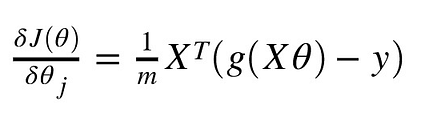# Logistic regression implementation with numpy¶

Implement logistic regression in python using batch gradient descent. Use your code to fit the data given above. Make sure you save the value of your loss function on each iteration in a data structure (e.g., list).

## Implementation details [writeup]¶

I have written a class that can do binary classification using logistic regression on a dataset with multiple features. It has 6 methods. These are listed and described below:

#### logistic_regr_hypothesis(self, X, theta)¶

This method takes an data set and theta values and dot products them together. This gives and array of values for a linear hypothesis. It runs the logistic function with this as input (see equation below).

$$h_ \theta (x) = \frac{\mathrm{1} }{\mathrm{1} + e^- \theta^Tx }$$

This returns a <class 'numpy.ndarray'> of probability values between 0 and 1. Closer to 1 means it is more likely to be a class 1 and if its closer to 0 its more likely to be 0.

#### loss_cross_entropy(self, h, y)¶

This method takes an array of hypothesis values (such as returned by logistic_regr_hypothesis(...)) and the ground truth values (ie. the actual class) and calculates the cross entropy cost; the mean of the cost values. It returns this value.

#### add_intercept(self, X)¶

This method creates a nump.ndarray of ones and concatenates this with the input array $X$ and transposes. This adds an element of value 1 to each input value which means when $\theta_ 0$ is multiplied by one. Example output below:

[[1.   2.75]
[1.   1.75]
[1.   1.5 ]
[1.   4.25]
[1.   1.75]
[1.   4.75]
[1.   5.5 ]
[1.   2.25]
[1.   0.5 ]
[1.   3.5 ]
[1.   4.  ]
[1.   3.25]
[1.   2.  ]
[1.   5.  ]
[1.   1.25]]


#### logistic_regr_fit(self, X, y):¶

This method fits input values with output values. Firstly it calls the add_intercept method for $X$ and initalises all $\theta_ i$ to 0.

self.theta = np.zeros(X.shape)


It then loops self.n times (number of iterations) and calls some methods. Firstly it calls the logistic_regr_hypothesis(self, X, theta) method to calculate the intial hypothesis values based on the $\theta_ i$. It then calculates the gradient of the loss values using a derivative of the loss function (see equation below) and updates theta using this gradient and the learning rate.self.theta = self.theta - grad*self.lr


It calcuates the loss_cross_entropy and then stores this. After the loop it returns final $\theta_ i$ values and the loss for each iteration.

#### predict_probabilities(self, X):¶

This predicts outputs based on a set of inputs using the trained model (using the optimised theta values). It returns a list of probablities between 0 and 1.

#### predict_class(self)¶

This final method simply rounds the above output so as to return the predicted class. In this binary classifier values will be either 0 or 1.

## Questions¶

### [question 1]¶

Using a learning rate of 0.1 the algorithm seems to converge after about 1000-1500 iterations.

### [question 2]¶

If alpha (the learning rate) is too large the loss value seems to oscillate to varying degrees. This is due to overshooting the minimum and then overshooting back and looping around the minimum. See figure below...

### [question 3]¶

Assume that you are applying logistic regression to the iris (flower) dataset, as in the previous assignment. Answer the following questions:

#### (a) How would your hypothesis function change in this case and why?¶

The current hypothesis function works and with n of features. As shown in code cell below this one...

#### (b) How would you utilize your implementation of logistic regression in order to perform (multi-class) classification on the iris dataset?¶

I would implement a logistic regression method that would loop over each of a number of given classes and for each class do fit a logistic regression model via gradient descent. Then I would combine the models to give a multi class classifier. Pseudocode below:

# Fit per class and save theta values
theta_per_class = []
for c in classes:
theta_per_class.append(self.logistic_regression_fit(c.X, c.y))

y_probs = []
# For each theta set (ie model)
for theta in theta_per_class:
# Find prediction probabilities and store
y_probs.append([self.predict_probabilities(X, theta))

y_prob = []
# For each set of probabilities
for i, y in enumerate(y_probs.T):
# Find max and save
y_probs.append(np.argmax(y))